• ГДЗ
• ЕГЭ
• Пересказы

# Упр.9 Appendix 1 МатематикаГДЗ Enjoy English 10 класс

9 Do you remember how to solve a system of linear equations? Study the example below to make sure about one of the ways.
1 Во-первых, мы выражаем Y через X в первом уравнении. Затем мы выносим Y за скобки во втором уравнении.

2 Мы пишем Y-эквивалент из первого уравнения во втором уравнении. Итак, мы имеем следующее:

3 Умножаем (4 - Х) на (1 + X) и записываем новое выражение в левой части уравнения. Правая сторона всё такая же.

4 После того, мы переносим всё в левую сторону, чтобы выражение приравнять к нулю.

5 Затем мы изменим знаки, чтобы сделать квадратное уравнение более обычным.

6 Для того чтобы решить квадратное уравнение применим квадратичную формулу, которая верна для любого квадратного уравнения.

Мы видим, что в нашем уравнении
«b» равно -3
«а» равно 1
и «с» равно 2
Таким образом, мы вставляем эти цифры вместо букв и получаем следующее.

7 Затем мы упростим правую часть.

8 Знак ± показывает, что X может принимать два различных значения. Нам нужно решить выражение для X1 и X2

9 Теперь мы знаем значение X1 и X2, и мы должны выяснить значения Y1 и Y2. Давайте выразим их через X1 и X2 (см шаг 2).
(Y 1 равно четыре минус двух и равно двум)
(У2 равно четыре минус один и равно трем)

10 Теперь мы видим, что система уравнений имеет два решения. Запишем оба из них по мере необходимости.
Решение: (2; 2) (1; 3)

9 Do you remember how to solve a system of linear equations? Study the example below to make sure about one of the ways.
1 First, we express Y through X in the first equation. Then we take Y out of the brackets in the second equation.
2 We write the Y-equivalent from the first equation in the second equation. So, we have the following:
3 We multiply (4 - X) by (1 + X) and write the new expression in the left-hand side of the equation. The right-hand side is still the same.
4 After that, we transfer everything to the left-hand side to make the expression equal to zero.
5 Then we change the signs to make the quadratic equation look more conventional.
6 To solve the quadratic equation we apply the quadratic formula which is true for any quadratic equation.
We see that in our equation
b is equal to -3
a is equal to 1
and c is equal to 2
So, we use these numbers instead of the letters and get the following:
7 Then we simplify the right-hand side.
8 The sign ± demonstrates that X can take two different values. We need to solve the expression for X1 and X2
9 Now we know the value of X1 and X2, and we need to find out Y1 and Y2. Let’s express them through X1 and X2 (see step 2).
(Y1 is equal to four minus two and is equal to two)
(Y2 is equal to four minus one and is equal to three)
10 Now we can see that the system of equations has two solutions. We write down both of them as necessary.
Solution: (2; 2) (1; 3)

С данным решебником также смотрят: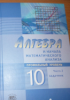## ГДЗ Мордкович (проф)10 класс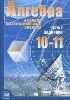## ГДЗ Мордкович10-11 класс## ГДЗ Мордкович10 класс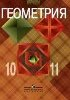## ГДЗ Атанасян 10-11 класс## ГДЗ Погорелов 10-11 класс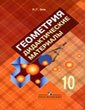## ГДЗ Зив 10 класс## ГДЗ Мякишев 10 класс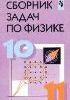## ГДЗ Степанова 10-11 класс## ГДЗ Касьянов 10 класс## ГДЗ Рудзитис 10 класс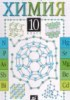## ГДЗ Гузей 10 класс## ГДЗ Семакин 10 класс## ГДЗ Габриелян 10 класс## ГДЗ Габриелян (проф)10 класс## ГДЗ Габриелян (РТ)10 класс

#### О сайте

Reshak.ru - самый полный сборник решебников для учеников старших классов. Здесь вы сможете найти решебники, ГДЗ, переводы текстов. Практически весь материал, собранный на сайте - сделанный для людей! Все решебники выполнены качественно, в понятном интерфейсе, с приятной навигацией. Вы сможете скачать гдз, решебник английского, улучшить ваши школьные оценки, повысить знания, получить намного больше свободного времени.
Главная задача сайта: помогать школьникам в решении домашнего задания. Кроме того, весь материал гдз совершенствуется, добавляются новые сборники решений, решебники по изучению английского языка.

#### Информация

©reshak.ru По всем вопросам обращаться на электронную почту: admin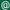reshak.ru. При копировании материала ссылка на сайт обязательна. Правообладателям